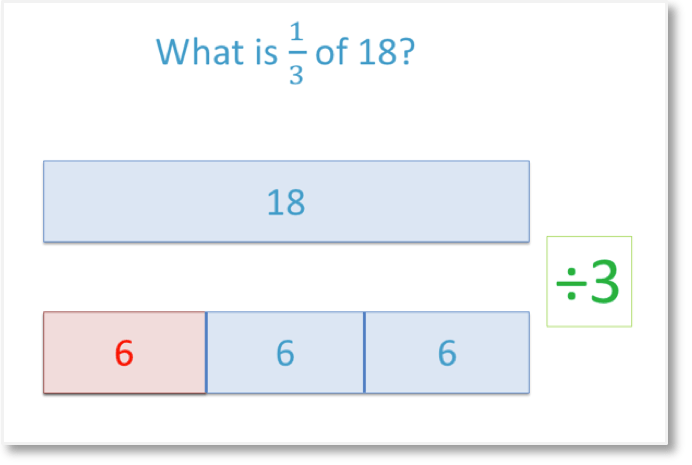# How to Simplify Fractions

How to Simplify Fractions• To simplify a fraction, divide the and by the same number.
• We notice that 2 and 6 are both in the two times table.
• Two is the that divides into both the numerator and denominator.
• We divide the top of the fraction by 2 to get '1'.
• We divide the bottom of the fraction by 2 as well to get '3'.
• The fraction   2 / 6   is simplified to   1 / 3.
• A fraction is fully simplified if it cannot be simplified any further.
To simplify a fraction, divide the numerator and denominator by the same number.• We simplify the fraction   24 / 60   by dividing the and by their .
• The highest common factor is 12, so we will divide the top of the fraction and the bottom of the fraction by 12.
• 24 ÷ 12 = 2 and 60 ÷ 12 = 5.
• The fraction   24 / 60   is simplified to   2 / 5.
• Alternatively, we can simplify our fraction in steps by dividing by any number that goes into both numbers.
• Both 24 and 60 are in the six times table so we can divide by six first.
•   24 / 60   simplifies to   4 / 10.
• However we can see that 4 and 10 are both even and can both be halved.
• The fraction is fully simplified to   2 / 5.
• We know that the fraction is fully simplified because there is no other number that divides into 2 and 5.
• We know this because 2 and 5 are both .# Simplifying Fractions

## What does it mean to Simplify a Fraction?

Simplifying a fraction means to write a fraction as an equivalent fraction with a smaller numerator and denominator. To simplify a fraction, divide the numerator and denominator by the same number. A fraction is fully simplified if it cannot be simplified any further.

For example, we will simplify the fraction   2/6  .

The numerator is the number on top of the fraction. The numerator is 2.

The denominator is the number on the bottom of the fraction. The denominator is 6.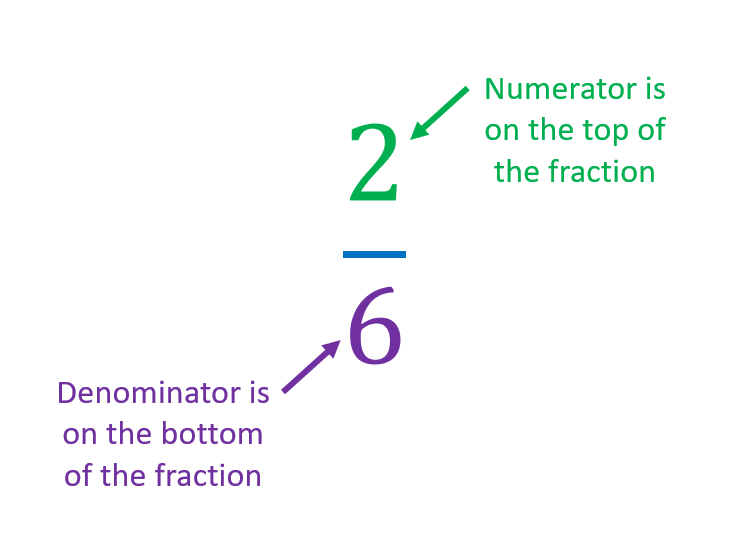To simplify a fraction, divide the numerator and denominator by the same number. The division must be exact because we cannot have a decimal number as the numerator or denominator.

2 and 6 are both even so we can divide them both by 2.To simplify a fraction, you must divide the numerator and denominator by the same value.

Since we have divided the numerator by 2, we also need to divide the denominator by 2.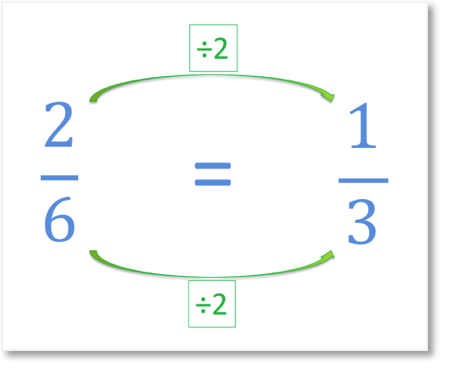2/6   simplifies to the fraction   1/3  .

A fraction written in its simplest form means that it cannot be simplified any further. This means that there is no equivalent fraction with a smaller numerator and denominator.

We cannot divide 1 and 3 by another number exactly. This means that the fraction of   1/ 3   is fully simplified.

The process of simplifying a fraction is also known as reducing a fraction.

Reducing a fraction to its lowest terms means that the fraction has been simplified fully. A fully reduced fraction does not have an equivalent fraction with a smaller numerator or denominator.

## How to Simplify Fractions Step-by-Step

To simplify a fraction fully, follow these steps:

1. Write all of the factors of the numerator and the denominator in two separate lists.
2. Divide the numerator by the largest number to appear in both lists.
3. Divide the denominator by the same number.

For example, fully simplify the fraction   24/60  .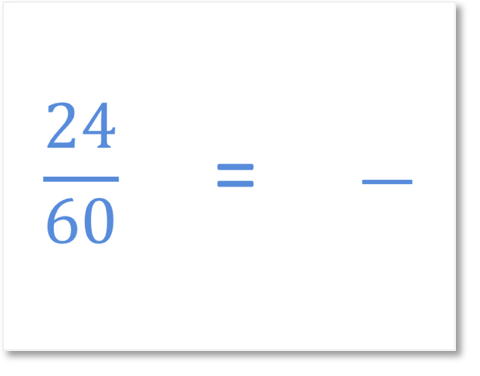The first step is to list all of the factors of the numerator and then list all of the factors of the denominator.The largest number in both lists is 12. 12 is the highest common factor (greatest common factor) of 24 and 60.

The highest common factor of two numbers is the largest number that can divide exactly into both numbers.

The next step is to divide the numerator by the highest common factor.

24 ÷ 12 = 2 and so, our new simplified numerator is 2.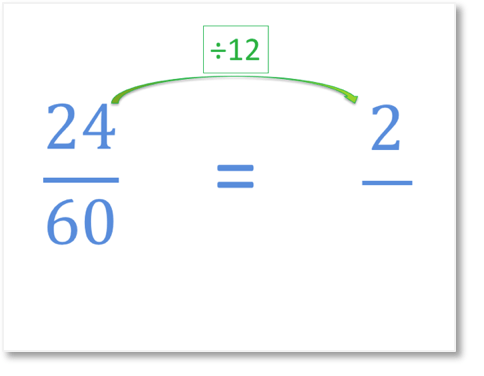The final step is to divide the denominator by the highest common factor.

60 ÷ 12 = 5 and so, our new simplified denominator is 5.24/60   fully simplified equals   2/5  .

To reduce a fraction to its lowest terms, divide the numerator and denominator by the greatest common factor.

No number, other than 1, will divide into both 2 and 5, so we have fully simplified the fraction into its simplest form.

The denominator is '5' which is a prime number.

We know a fraction is fully simplified if both the numerator and denominator are prime numbers. A fraction is fully simplified if the denominator is a prime number and the numerator is a smaller number than this. These are not the only ways to know if a fraction is fully simplified but they are two useful checks.

We can also see that the numerator can only be divided by 2 and that the denominator of '5' is odd and therefore cannot be divided by 2.

## Why do we Simplify Fractions?

Fractions are simplified to make calculations easier. The simplified fraction is the same value as the original fraction but it has smaller numbers. Smaller numbers are easier to work with.

For example, here is the fraction   150/350  .

If you had to multiply or add this fraction to another, the numbers would be quite large and could take longer to work out than if looking at smaller numbers.

A fraction does not need to be fully simplified in one step. Several steps can be used to fully simplify a fraction by dividing by any factor of both the numerator and the denominator each time.

We can see that both 150 and 350 end in a zero. This is a big clue that both numbers are divisible by 10.

We will simplify the fraction   150/350   to   15/35   by dividing the numerator and denominator by 10.The fraction has been reduced but it can be reduced further with another step.

Both 15 and 35 end in a 5, which tells us that both numbers are divisible by 5.

We will simplify the fraction   15/35   to   3/7   by dividing the numerator and denominator by 5.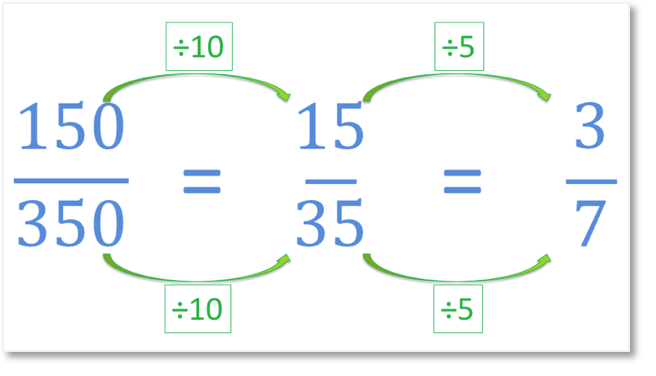We can see that the fraction is now reduced to its lowest terms because both 3 and 7 are prime. They cannot be divided by a number apart from one and themselves. There is no number that divided exactly into both 3 and 7 to make them smaller.

We can see that the fraction   3/7   is much simpler to use than   150/350  .

We reduce fractions to their lowest terms because it is easier to appreciate their size and compare them. It is also easier to use reduced fractions in calculations.

For example   3/7   is easier to visualise than   150/350   even though they represent the same number.

## How to Simplify a Mixed Number

To simplify a mixed number, follow these steps:

1. Leave the whole number part the same.
2. Find the greatest common factor of the numerator and the denominator.
3. Divide the numerator and denominator by the greatest common factor.
4. Write the simplified fraction immediately after the whole number part.

To simplify a mixed fraction, only simplify the fraction part. Leave the whole number part the same.

For example, simplify 5   2/4  .

The first step is to leave the whole number part the same. 5 remains as 5.

We can simplify   2/4   to   1/2   by dividing both the numerator and the denominator by 2.To simplify the mixed number 2   9/15  , we leave the whole number part the same and simplify   9/15  .

We write down the 2 and simplify   9/15   to   3/5   by dividing the numerator and denominator by 3.

The mixed fraction of 2   9/15   simplifies to 2   3/5  .

## How to Simplify an Improper Fraction

To simplify an improper fraction, simply divide the numerator and denominator by their greatest common factor. Simplify an improper fraction in exactly the same way as simplifying a proper fraction.

An improper fraction behaves in the same way as a proper fraction. An improper fraction is simply a fraction with a larger numerator on the top than its denominator on the bottom.

For example, simplify the fraction   20/8  .

The greatest common factor of 20 and 8 is 4. 4 is the largest number that divides exactly into both 20 and 8.

The improper fraction of   20/8   simplifies to   5/2   when the numerator and denominator are both divided by 4.Here is the example of reducing the improper fraction of   42/18  .

The greatest common factor of 42 and 18 is 6. 6 is the largest number that divides exactly into both 42 and 18.

We divide the numerator and denominator by 6 to simplify   42/18   to   7/3  .

When we simplify an improper fraction, the answer is still an improper fraction.Now try our lesson on Unit Fractions of Amounts where we learn what unit fractions are and how to calculate unit fractions of amounts.